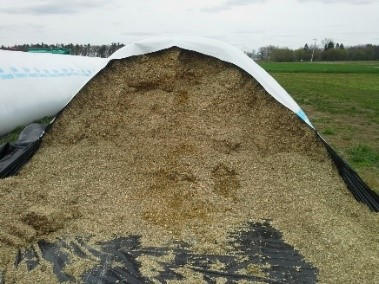# Determining the Tons of Silage in Silo Bags for CFAPKevin Jarek, Liz Binversie, Bill Halfman, Paul Mitchell

This tool helps a farmer calculate the tons of silage in bunker silos and convert it to its grain equivalent for including in 2019 production for determining CFAP payments.

Step 1: In the yellow cells, enter the Silage Dry Matter Content, Dry Matter Density, Bag Diameter, and Silage Length.

• Silage Dry Matter Content is typically 35% meaning wet silage is 65% moisture.
• The DM density of the feed ranges from 11-15 lbs of DM per cubic foot. Densities will vary based on forage moisture, length of cut, and many other factors. A value of 13 lbs DM per cubic foot is often used as an average value.
• The Silage Length equals Bag Length – (Plastic Length – 2 x Diameter). For example, the silage length for a 10 ft bag that is 150 long is 150 – (2 x 10) = 130 ft.
• For a bag that was partially fed on January 15, 2020, reduce this value by the length of bag that had already been fed.

Step 2: In the pink cells, read Tons Dry Matter.

Step 3: Divide Tons Dry Matter by 0.35 to convert silage tons of DM to standard 65% moisture.

Step 4: Multiply by the FSA conversion factor. Corn silage is 7.94 bu/ton (at 65% moisture) and 4.08 bu/ton (at 65% moisture) for oatlage.

Example: Determine the wet tons of 65% silage in an 8 ft X 200 ft silo bag.

1. Enter Silage Dry Matter Content Start of 0.35 (35%), DM Density of 13, Bag Length – (Plastic Length – 2 x Diameter) = 200 – (2 x 8) = 184 ft (16 ft lost tying off both ends of the bag), and Bad Diameter of 8.
2. Spreadsheet calculates 60.12 Tons Dry Matter in pink cell.
3. Divide by 0.35 to convert to standard 65% moisture: 60.12/0.35 = 171.8 tons wet silage.
4. Convert to grain equivalents using FSA conversion factors:
• Corn Silage: 172 x 7.94 bu/ton = 1,366 bu of Corn
• Oatlage: 172 x 4.08 bu/ton = 702 bu of Oats
• Soybean Silage: 172 x 5.00 bu/ton = 860 bu of Soybeans
• Grain Sorghum Silage 172 x 5.56 bu/ton = 956 bu of Sorghum